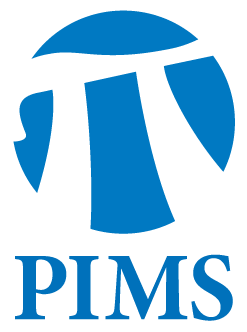## UBC Colloquium and PIMS Distinguished Guest Lecturer: Thomas Scanlon

• Date: 01/06/2012
• Time: 15:00
Lecturer(s):
Thomas Scanlon
Location:

University of British Columbia

Topic:

A logician's view of diophantine geometry

Description:

Abstract

To a logician diophantine geometry, the study of geometric relations on
points of arithmetic origin, is almost a contradiction in terms since
geometrical reasoning has long been known to be tame and decidable (from
the 1929 work of Tarski on elementary geometry) while by Gödel's
incompleteness theorems arithmetic is known to be infected by inherent
undecidability.  However, this tension has not prevented geometers and
number theorists from investigating a subject they know to be hard but
one in which ideas from geometry explain the apparent regularity of the
solutions in rational numbers, roots of unity, or other arithmetically
interesting to certain geometrically constrained systems of equations.
In many important cases, model theorists can explain or prove such
theorems by showing that the structure on the arithmetic points simply
reflects the tameness of the class of definable sets in some structure
intermediate between algebraic geometry and arithmetic.

With this lecture I will broadly survey the project of applying the
model theory of enriched, though still tractable, geometries such as
those coming from differential or difference algebra, to number
theoretic problems such as those around the conjectures of Mordell-Lang
and André-Oort or arising from arithmetic dynamics.

Other Information:

Location: MATX 1100